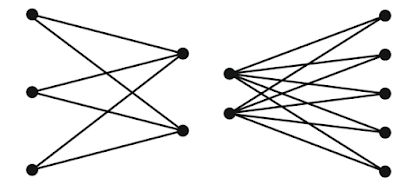Different types of graphs in data structures

A graph G consists of two sets:

(i) A set V, Called the set of all vertices( or nodes)

(ii) A str V, called the set of all edges. This set E is the set of all pairs of elements from V.

1. Directed Graph: A digraph is also called a directed graph. It is a graph G, where each edge have a direction. In the above figure, graph G2 is a directed graph.

2.  Undirected graph: An undirected graph G is a graph in which each edge e is not assigned a directed. In the above figure, graph G is a undirected graph.

3. Connected graph: A graph ( not digraph) is called connected if there is a path from any vertex to any other vertex.

A graph G is connected if and only if there is a simple path between any two nodes in G. A graph G is said to be complete if every node U in G is adjacent to every other node V in G. A complete graph with n nodes has: n*{(n-1)/2} edges.

4. Weighted graph: A weighted graph is a graph in which edges are assigned weights. This is often required to model certain physical situations by means of a graph.

5. Complete graph: A graph (digraph) G is said to be complete if each vertex Vi is adjacent to every other vertex Vj in G. In other word, there are edges from any vertex to all other vertices.

6. Null Graph- A graph which contains only isolated node is called a null graph i.e. set of edges in a null graph is empty.

7. Regular Graph: A graph in which all the vertices are of equal degree is called a regular graph. If the degree of each vertex is r, then the graph is called a regular graph of degree r.

Every null graph is a regular graph of degree zero and a complete graph Kn is a regular graph of degree n-1.

8. Cyclic Graph - A graph with continuous sequence of vertices and edges is called a cyclic graph.
Cyclic graph is denoted on ‘n’ vertices bt Cn where n >=3.

9. Wheel Graph - A graph formed by adding a vertex inside a cycle and connecting it to every other vertex is known as wheel graph.

Wheel graph is denoted on 'n' vertices bt Wn where n>=3.

10. Bipartite Graph- A graph G=(V,E) ia bipartite if the vertex set V can be partitioned into two subsets V1 and V2 such that every edge in E connects a vertex in V1 and a vertex in V2 (no edge in G connects either two vertices in V1 or two vertices in V2) is called a bipartite graph. A bipartite graph can have no loop.

11. Complete Bipartite Graph - A graph where a set of vertices of a graph can be partitioned into two subsets in such a way that no pair of vertices in the same set are adjacent to each other and every vertex of first set is adjacent to the second set.More

More

More

More

More

More
Top